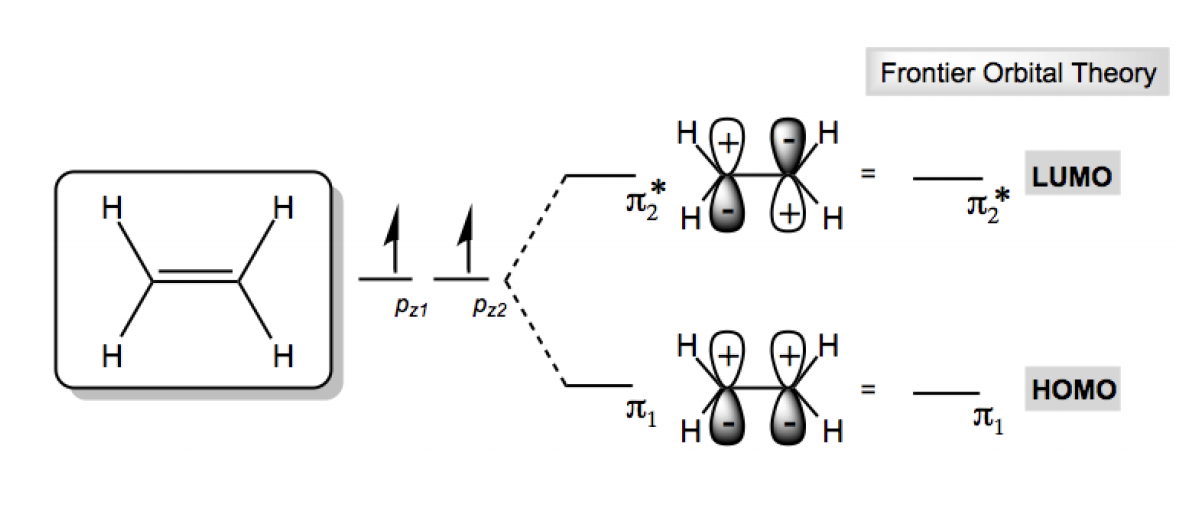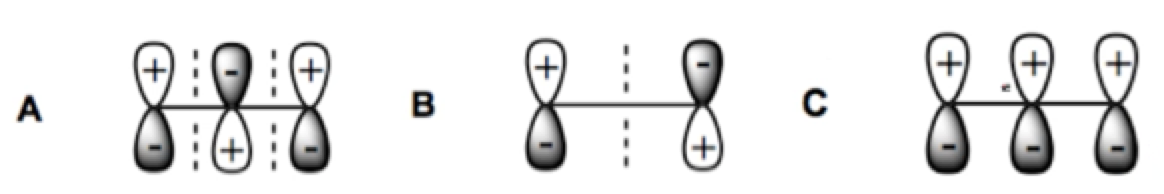## Organic Chemistry

Learn the toughest concepts covered in Organic Chemistry with step-by-step video tutorials and practice problems by world-class tutors.

16. Conjugated Systems

# HOMO LUMO

Frontier orbital interations are the driving force behind many Organic Chemistry recations. Let's find out why.

1
concept

## HOMO vs. LUMO2m
Play a video:
Hey, everyone, In this video, I want to introduce a really important concept in organic chemistry called Frontier Molecular orbital theory. Let's take a look. Frontier Orbital interactions are actually the driving force behind many reactions in organic chemistry. So this information that we're gonna learn now is actually gonna be foundational toe understand reactions later. So it's really important that we know what this F mot theory is. Okay. And the most important thing that we need to know about frontier molecular orbital's is how toe find homo and limo. So you might have heard about homo and limo before, but in this lesson, we really need to fully understand it. So the homo is to find as the highest occupied molecular orbital out of all your molecular orbital's. It's the one that has the highest energy that still has electrons in it. Okay, then there's the limo. The limo is the lowest unoccupied molecular orbital. So once you get out of all of your molecular orbital's, it's the one that has the lowest energy while still having no electrons in it. Okay, so if we were to look at eth een as an example, so ethane is a very simple molecule, so it's easy to understand home a limo. So what we would have is we have two atomic orbital's right and those two atomic orbital's form to molecular Orbital's. We have the bonding orbital. We have the anti bonding orbital and we know that according to the principles of electron configuration, thes two electrons should Onley fill the bottom pie. One orbital. It's called pie one, not Sai one, because it's just a double bonds. You just called up. I won and that means pie to star, which is our anti bonding. Orbital is unfilled. Okay, so what that means is that our homo orbital is pie one. So we would say that the homo contains two electrons. It's pie one, and that means that my loom Oh, orbital is pie, too. And it has zero elections. That's it. So Homo is the highest one that still has electrons, which in this case it's the Onley one, because it's the only one that exists, and Lou Mo has a no electrons in it, and it's the lowest energy state. But since there are only two, which just happens to be the only one that doesn't have electrons as your molecules get bigger and the number of molecular orbital increases finding home one limo will be on that basically the cusp of where the electrons end and where the unfilled orbital's begin. So let's go ahead and try out a practice problem.
• HOMO = Highest Occupied Molecular Orbital
• LUMO = Lowest Unoccupied Molecular Orbital2
Problem

Consider the MO's of allyl anion. Which of the following are HOMO and LUMO?